If you have a related question, please click the "Ask a related question" button in the top right corner. The newly created question will be automatically linked to this question.

# TLV2372: How to step down the output voltage of the filter to 0 to 3V from 0 to 10V keeping same cut off frequency (60KHz) at -3dB and order (3rd order Butterworth filter)?

Part Number: TLV2372

Hi,

• The input to the circuit given below is a sine wave with amplitude 5V (0V to 10V) and frequency 1.4k Hz.
• It is a 3rd order Butterworth filter with cut off frequency of 60KHz at -3dB
• Here I am getting the output of 5V (0V to 10V) with cut off frequency of 60KHz at -3dB

Problem statement:

• How to step down the output voltage of the filter to 0 to 3V from 0 to 10V keeping same cut off frequency (60KHz) at -3dB and order (3rd order Butterworth filter)?• Hi Mahima,

replace the 2k67 resistor by a voltage divider Rx / Ry with

(Rx + Ry) / Ry = 10 / 3

and

1 / Rx + 1 / Ry = 1 / 2k67

Kai

• Hi,

Am unable to get the cut off frequency of 60KHz at -3dB when I followed the steps you mentioned.

So, it would be very helpful if you provide the exact value and circuit of resistive divider network and filter for an output cut off frequency of 60KHz at -3dB and output voltage of 3V (Input is 1.4KHz with 0 to 10V sine wave).

Note: Please provide the above information with the help of circuit.

Thank you.

• Hi,

Adding to the info what I have mentioned:

I have done the changes as mentioned in your response still not getting the desired output.

Circuit: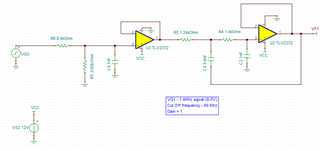Frequency response: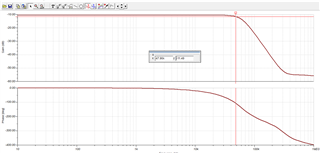Transient response: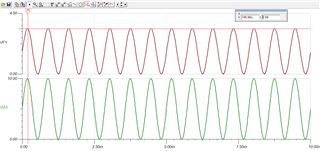Thank you.

• Mahima,

-3dB point looks close to 60kHz to me. The divider attenuates the input signal with a ratio of .3V/V ( -10.4 dB), therefore the -3dB point would be at -13.4 dB on the frequency response curve: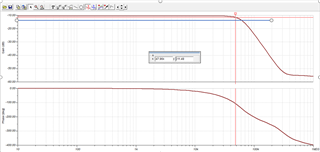Recall, the restive divider should not be changing the filter response so long as the equivalent resistance of R1||R6 = R1_S1

Please let me know if you have any questions,

Best,

Jacob

• Thank you so much.

• Hi Mahima,

works for me: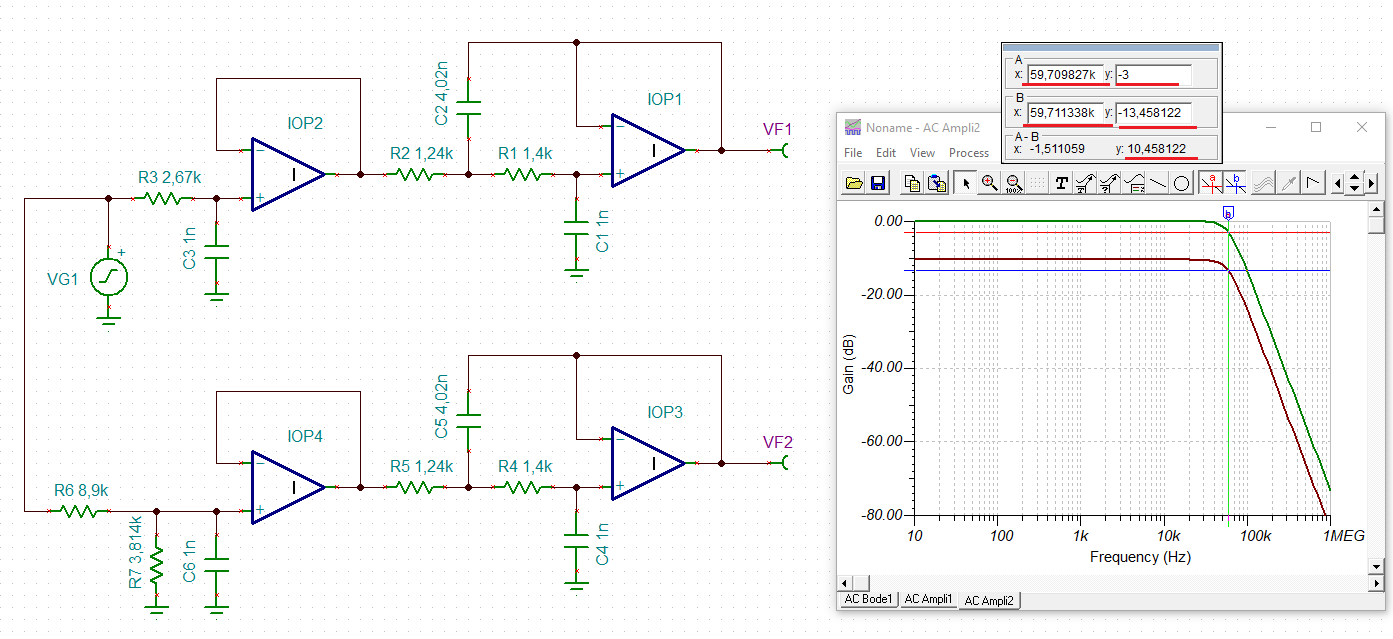mahima_low_pass.TSC

Kai

• And for the TLV2372 as well: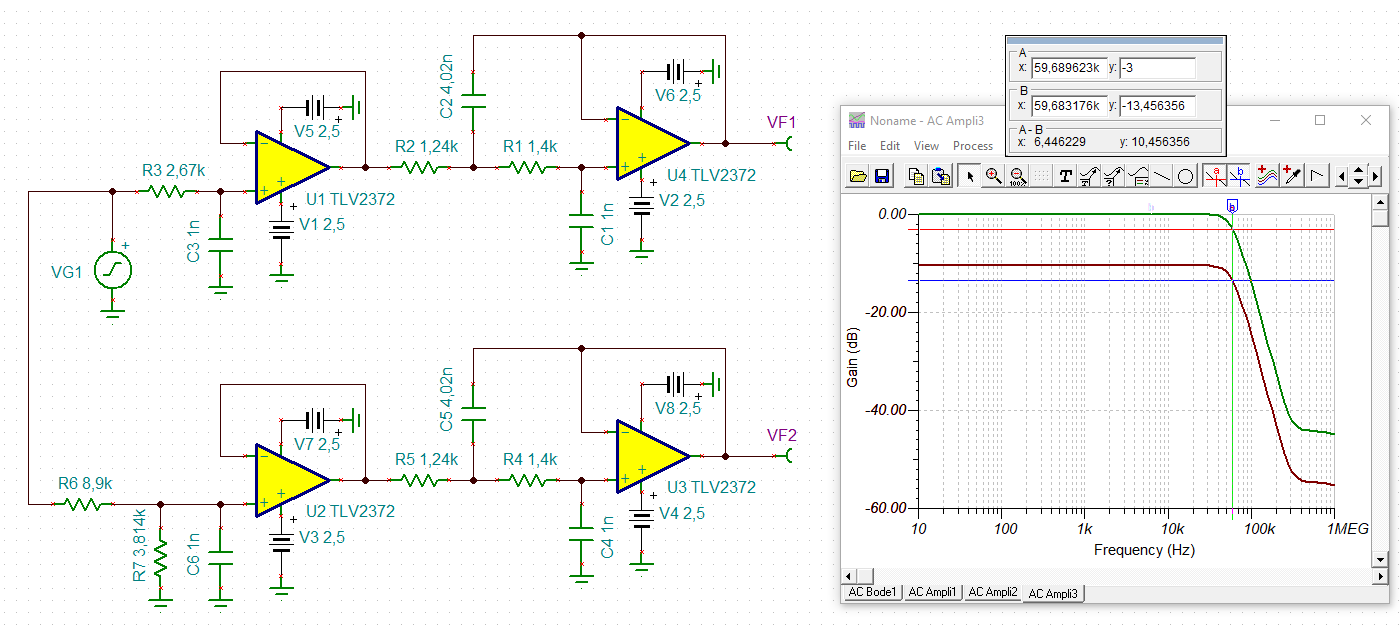mahima_tlv2372.TSC

Kai